# Archives in Chemical Research Open Access

• ISSN: 2572-4657
• Journal h-index: 5
• Journal CiteScore: 1.16
• Journal Impact Factor: 1.45
• Average acceptance to publication time (5-7 days)
• Average article processing time (30-45 days) Less than 5 volumes 30 days
8 - 9 volumes 40 days
10 and more volumes 45 days
Reach us +32 25889658

Research Article - (2018) Volume 2, Issue 1

## Symmetry of Gold Neutral Clusters Au3-20 and Normal Modes of Vibrations by using the Numerical Finite Difference Method with Density-Functional Tight-Binding(DFTB) Approach

Vishwanathan K*

Physical and Theoretical Chemistry, University of Saarland, 66123 Saarbrücken, Germany

*Corresponding Author:
Vishwanathan K
Physical and Theoretical Chemistry,
University of Saarland, 66123
Saarbrücken, Germany.
Tel: +49-0151-63119680
E-mail: vishwa_nathan_7@yahoo.com

Received date: September 05, 2017; Accepted date: October 25, 2017; Published date: November 01, 2017

Citation: Vishwanathan K (2017) Symmetry of Gold Neutral Clusters Au3-20 and Normal Modes of Vibrations by using the Numerical Finite Difference Method with Density-Functional Tight-Binding (DFTB) Approach. Arch Chem Res. Vol.2 No.1: 4. doi: 10.21767/2572-4657.100017

Copyright: © 2017 Vishwanathan K, et al. This is an open-access article distributed under the terms of the Creative Commons Attribution License, which permits unrestricted use, distribution, and reproduction in any medium, provided the original author and source are credited.

Visit for more related articles at Archives in Chemical Research

#### Abstract

The geometries and vibrational frequency of the most stable small AuN clusters with N = 3 to 20 are presented through global structure re-optimization Study. The finite-differentiation method has been implemented within the density-functional tight-binding (DFTB) approach. The desired set of system eigenfrequencies (3N-6) is obtained by a diagonalization of the symmetric positive semi definite Hessian matrix. We have observed the vibrational modes between 0.55 and 370.72 cm-1 in wavenumbers at T=0 for the small gold clusters. The effect of the range of interatomic forces was calculated, and even the very lower frequencies were occupied in some clusters.

#### Keywords

Gold Atomic Clusters; Density-Functional Tight-Binding (DFTB) approach; Finite-Differentiation Approximation; Force Constants (FCs); Normal Modes of Vibrations

#### Introduction

Gold is an important material (resistant to most acids, used in infrared shielding, colored-glass production, gold leafing, and tooth restoration as well as a good conductor of heat and electricity due to that it was attracted a great attention) and an outstanding landmark in cluster science. Small clusters often will have a different physical and chemical properties than their bulk ones. Particularly, small particles of gold differ from the bulk as they contain edge atoms that have low coordination and can adopt binding geometries which lead to a more reactive electronic structure [1,2].

The study of nanostructured materials exhibiting novel properties is one of the most fascinating fields of current research. Small nanomaterials are of particular interest because of intriguing characteristics [3-6]. Nanoparticles with smaller dimensions may exhibit different properties in comparison with bulk material.

The nanoparticles possess unique physico-chemical, opticaland biological properties which can be manipulated suitably for desired applications . Particulary, gold chemistry plays a very important role in nanoelectronics and bionanosciences .

In the present work, we apply a parameterized density functional tight-binding method combined with an numerical differentiation-finite difference method on gold clusters with from 3 to 20 atoms. We have extracted the normal modes of vibration and the respective frequencies of the clusters, classified according to their symmetry group. However, we only study on the behaviour of the vibrational spectrum which are existed in small neutral gold clusters at low-temperatures, T=0. In addition, we provide computational evidence of the existence of the novel properties of the interatomic interaction energy of the atoms within the clusters.

#### Theoretical and Computational Procedure

The DFTB9–11 is based on the density functional theory of Hohenberg and Kohn in the formulation of Kohn and Sham. In addition, the Kohn-Sham orbitals Ψi(r) of the system of interest are expanded in terms of atom-centered basis functions(1)

While so far the variational parameters have been the realspace grid representations of the pseudo wave functions, it will now be the set of coefficients cim. Index m describes the atom, where fm is centered and it is angular as well as radially dependant. The fm is determined by self-consistent DFT calculations on

isolated atoms using large Slater-type basis sets. In calculating the orbital energies, we need the Hamilton matrix elements and the overlap matrix elements. The above formula gives the secular equations(2)

Here, cim’s are expansion coefficients, ei is for the single-particle energies (or where ei are the Kohn-Sham eigenvalues of the neutral), and the matrix elements of Hamiltonian Hmn and the overlap matrix elements Smn are defined as(3)

They depend on the atomic positions and on a well-guessed density r(r). By solving the Kohn-Sham equations in an effective one particle potential, the Hamiltonian ˆH is defined as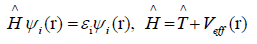(4)

To calculate the Hamiltonian matrix, the effective potential Veff has to be approximated. Here,being the kinetic-energy operatorand Veff (r) being the effective Kohn-Sham potential, which is approximated as a simple superposition of the potentials of the neutral atoms,(5)

is the Kohn-Sham potential of a neutral atom, rj = r-Rj is an atomic position, and Rj being the coordinates of the j-th atom. The short-range interactions can be approximated by simple pair potentials, and the total energy of the compound of interest relative to that of the isolated atoms is then written as,(6)

Here, the majority of the binding energyis contained in the difference between the single-particle energies Ɛi of the system of interest and the single-particle energiesof the isolated atoms (atom index j, orbital index mj),is determined as the difference betweenandfor diatomic molecules (withbeing the total energy from parameter-free densityfunctional calculations). In the present study, only the 5d and 6s electrons of the gold atoms are explicitly included, whereas the rest are treated within a frozen-core approximation [9-12].

#### Structure of the Gold Atomic Clusters

Initially, a study on the structural and electronic properties of the global minimum gold cluster structures was obtained by combination of a genetic algorithm together with DFTB energy calculations . Dong and Springborg study explicitly includes the electronic degrees of freedom. This feature turned out to be crucial since the electronic properties of the gold clusters seem to play an important role in the determination of their structure. When including orbital interactions, packing effects as well as directional interactions determine the cluster structure. Packing effects lead to high symmetry structures and to the so-called magic numbers. Including orbital interactions in the calculations leads to a partial suppression of the magic-numbers and to low-symmetry structures. In most other metals, packing effects are predominant. On the other hand, the structures of most covalent molecules are determined by orbital interactions. For gold clusters, both seem to be important. Springborg and Dong suggest, that it is exactly this competition between packing effects and directional interactions which leads to low-symmetry clusters.

In their study, Dong and Springborg found the transition from planar to three-dimensional structures for too small cluster sizes N [14,15]. This could eventually be explained by the use of the parametrized DFTB method. However, low-symmetry structures have been found in other, more accurate studies on selected cluster-sizes, too. The comparison of their results with results from spherical-jellium-model calculations revealed some important differences. The stability-function, which gives information about particularly stable and unstable structures has much lower oddeven amplitudes compared to the stability function obtained within the jellium-calculations. This can be attributed to the lower symmetry. The structures do not resemble to fragments of crystalline gold-phases. Nevertheless, some regular patterns are found, e.g. the clusters with size up to 20 atoms are built up of atomic shells

#### Molecular Vibrations

In this section, we are going to introduce the mathematical formalism on which the developed method is based. We will see that, treating the problem within the so called normal-modeharmonicoscillator (NMHO) approximation , the introduction of the Hessian matrix and its diagonalization ultimately leads to the eigenfrequencies of the system and its eigenvectors, describing the harmonic motion of the clusters atoms .

Small vibrations in classical mechanics

Let us consider a stable structure consisting of N atoms. Let xa;ya and za be the coordinates of the ath atom of the structure and aa;ba and ca the values of the equilibrium positions of the ath atom. Displacements from the equilibrium positions can then be expressed by Δxα = (xα-aα); Δyα = (yα-bα); Δzα = (zα-cα). In the above notation, the classical kinetic energy T of the structure is given by(7)

It is customary to express the coordinates Δx1; :::; ΔzN by a new set of so called mass weighted coordinates q1; :::;q3N, defined as follows(8)

In terms of the time derivatives of these coordinates, the kinetic energy is(9)

NMHO approximation and re-optimization

We start out with structures that have been optimized . That means that we have found the lowest total energy and that the forces on the atoms are vanishing. We may then write the total energy in a Taylor series around the optimized structure, so the total energy depends on the positions of the atoms relative to each other and therefore depends on the q’s. For small displacements, the total energy E may be approximated as a Taylor series in q, within the actual normal-mode-harmonic-oscillator (NMHO) approximation, the cubic and higher order terms are neglected in the Taylor series, where the coefficients fi and fij are given by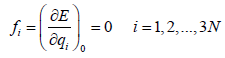(10)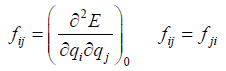(11)

This procedure implies a few customary consequences on which the popularity of the NMHO-model is based. First of all, since the energy has become quadratic, the vibrational motion we get solving Newton’s equations of motion will be harmonic. The further formulation of the problem leads to the Hessian matrix of the system, which allows us a simple analysis of the vibrational motion of the observed system. In the chosen notation, Newton’s equation of motion can be cast in the form(12)

we get, the equation determining the motion of the coordinates(13)

This is a set 3N simultaneous second-order linear differential equations. A possible solution is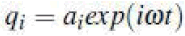and derivation with respect to time yields toand can be written as(14)(15)

here, δij is called the Kronecker delta.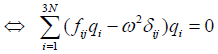(16)(17)

Dividing byleads to a set of 3N algebraic equations: Introducing matrix notation, we get(18)

where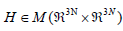is the Hessian matrix containing the second partial derivatives of the total energy of the system with respect to the structure (mass-weighted) coordinates of the nuclei. E being the total energy of the molecule/cluster, i.e. the single point energy. I is the identity operation in,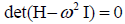is a column vector containing the amplitudes ai and theare the frequencies.

Equation (12) has non-vanishing solutions only, if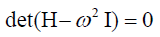(19)

Hence, the frequencies are obtained by consulting the roots of the characteristic polynomial, i.e., the frequencies are obtained by finding the eigenvalues of the Hessian matrix H.

In the case of a structure situated at a minimum on the energy surface the Hessian matrix should be symmetricpositivesemidefinite and therefore hermitian. It can be shown that the eigenvectors of an hermitian matrixconstitute an orthonormal basis (onb) of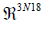. The representation of the Hessian matrix in this basis is diagonal and the diagonal elements are the sought eigenfrequenciesMoreover, the corresponding eigenvectorsof H are the directions of the harmonic motions of the molecule. They constitute the so-called normal modes. To fully specify any N-atomic structure or its vibrational motion, only ((3N-5) for linear and (3N-6) for non-linear) basis-vectors are needed. The five/six remaining basis vectors span Kernel of the Hessian, i.e., they determine the absolute position and orientation of the molecule in the inertial system. Thus, there are only ((3N-5) for linear and (3N-6) for non-linear) linearly independent vibrations, the molecule can undergo. From the construction of H, it follows that the molecules motions along the normal modes are harmonic oscillations. Moreover, since we are in a linear approximation, every possible harmonic motion of the molecule can be written as a linear combination of the normal modes.

The finding of the frequencies of the normal modes of the clusters involves an eigenvalue problem which can be solved by diagonalization of the symmetric positive semidefinite Hessian matrix of the system. So, in the present study, two different numerical diagonalization methods have been applied. The first method bases on Jacobi-transformations of the symmetric Hessian. The second method relies on the Househoulder-reduction of the symmetric Hessian to a tridiagonal form and subsequent application of a QLalgorithm which yields the eigenvalues and vectors. Both methods are standard procedures and a complete and comprehensive description is given in the Numerical Recipes, both methods were implemented in the main-program unit without any modifications with respect to the subroutine-codes presented [18,19]. Within the numerical error, both methods yield the same results.

The normal mode harmonic oscillator (NMHO) approximation

Please note, that from now on, for convenience, the total energy E of equation 6 in section 2 has the meaning as a potential energy surface V. The potential energy depends on the positions of the atoms relative to each other and therefore depends on the q’s. For small displacements, the potential energy V may be approximated as a Taylor series in q.

Now, we express the potential energy as a Taylor series.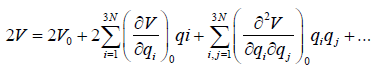(20)(21)

and with(22)

W. l. o. g., V0 can be set to zero. And since the qi’s are the distances from the equilibrium positions, the potential energy must have a minimum at

{qi = 0 | i = 1,2,.., 3N}, (23)

and we get(24)

Within the actual normal-mode-harmonic-oscillator (NMHO) approximation, the cubic and higher order terms are neglected in the above series, such that the energy expression (19, 20) becomes(25)

where the coefficients fij are given by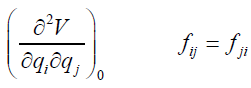(26)

This procedure implies a few customary consequences on which the popularity of the NMHO-model is based. First of all, since the potential has become quadratic, the vibrational motion we get solving Newton’s equations of motion will be harmonic. The further formulation of the problem leads to the Hessian matrix of the system, which allows us a simple analysis of the vibrational motion of the observed system.

#### Numerical Differentiation: Finite Difference Method

For the purpose of the present study, a very high accuracy of the optimum structures is required. In a first approach, we attempted to re-optimize the structures using the same steepest-descent method which has already been used for the first optimization . The vibrational properties are treated within the normal-mode harmonic oscillator model . Therefore, we needed to find a suitable scheme to set up the Hessian matrix of the system. Since we were using densityfunctional tight binding energy calculations, we do not have an analytical expression for the energy. Thus, we have to rely on numerical differentiation. Subsequently, we had to test the results in order to find suitable sets of differentiation parameters, i.e., suitable combinations of differentiation step-size and order of the polynomial. In order to get clusters which are somewhat closer to the actual minimum on the potential energy surface, we turned to a new strategy. This new strategy relies on the properties of the Hessian eigenvectors. Based on the assumption, that in the proximity to a minimum, the curvature of the potential energy surface does only change very little, we developed a method which could actually cope with the high numerical accuracy of the local structure optimization. Briefly, this method is described as follows: The Hessian matrix is represented in an orthonormal basis consisting of the six eigenvectors of the Hessian matrix which span its kernel and of (3N-6) arbitrarily chosen mutually orthonormal basis vectors, which are orthogonal to the kernel-eigenvectors. When represented in this basis, the Hessian should be partially diagonal. The diagonal part is now cut away and the remaining Hessian is diagonalized to reveal the eigenfrequencies of the clusters normal modes. Through the obtained eigenfrequencies, it is possible to set up the vibrational partition function of the examined systems, which gives access to the sought thermodynamic properties. The Hessian matrix is the matrix of second derivatives of the energy with respect to geometry which is quite sensitive to its geometry. Energy second derivatives are evaluated numerically. Themmass-weighted Hessian matrix is obtained by numerical differentiation of the analytical first derivatives, calculated at geometries obtained by incrementing in turn each of the 3N nuclear coordinates by a small amount ds with respect to the equilibrium geometry. The introduction of the Hessian matrix and its diagonalization ultimately leads to the eigen-frequencies of the system and its eigenvectors, describing the harmonic motion of the clusters atoms. In order to obtain the matrix elements Hi j of the Hessian matrix which are needed if one wishes to investigate the clusters thermodynamic properties and one should obtain the derivatives of potential energy surface (PES).

Cutting off the kernel

The Hessian matrix H is symmetric by Schwarz’ theorem. The Kernel of H consists of all vectors which describe pure translational and rotational motion of the center of mass of the molecule, leaving its internal structure untouched. This is the eigenspace of H which is associated to the eigenvalue 0. As we have 5 for linear 6 for non-linear degrees of freedom corresponding to such translations and rotations, dim(Ker(H)) = 5/6. We denote the five/ six Hessian eigenvectors associated to the Kernel. The remaining (3N-5)/(3N-6) Hessian eigenvectors denoted by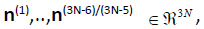form a basis of the (3N-5)/(3N-6) dimensional configuration space, in which the molecular structure may be described uniquely without any reference to the position or orientation of the molecule relative to an inertial system. In our new method, we apply the Gram-Schmidt theorem, to set up an orthonormal basis for. The basis of the Kernel consisting of the five/six hessian eigenvectors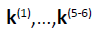can easily be found, they are the orthonormalized translations and rotations of the structure. Now, we simply useas the first five/ six basis vectors for an orthonormal basis ofdenoted C. The remaining (3N- 5)/(3N- 6) basis vectors of C are the arbitrarily chosen mutually orthonormal vectors, which have to satisfy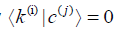for any possible combination of i and j.

By construction, the basis vectors c(1),…,c(3N-5)/(3N-6) of basis C form a basis of the (3N-5)/(3N-6) dimensional configuration space which is the subspace of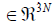configuration space, the normal modes n do not contain any components of the basisof the kernel of the Hessian. Thus, the normal modes nsatisfying the conditioncan be expanded in the basis c(1),…,c(3N-5)/(3N-6) of the configuration space and they will still be orthonormal. Now, let us represent H in the basis C. Letbe the matrix consisting of the column vectors of C, i.e.,(27)

Since U is a unitary transformation, the complex-conjugate of U is equal to its inverse, i.e., U* =U-1 and thus the sought representation H’ of H in the new basis C is found by calculating U*HU = H’ Since the first five/six vectors basisof the basis C are the eigenvectors corresponding to translation and rotation, the first five/six lines and columns of the representation H’ of H in this basis should be diagonal and the eigenvalues which are the H' ’s diagonal elements should be equal to 0.

Diagonalization of the non-diagonal submatrix H’’ Ɛ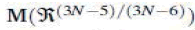which is the representation of the Hessian in the basis c(1),…,c(3N-5)/(3N-6) of the configuration space, yields its eigenvectors, i.e., the (3N-5)/(3N-6) normal modes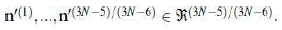The diagonal elements are the sought eigenvalues, the eigenfrequencies of the normal modes which are needed for the calculation of thermodynamic properties.

First, we set up an orthonormal basis which allows to separate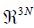into its (3N-5)/(3N-6)-dimensional configuration subspace and the complementary five/six dimensional subspace which makes reference to absolute position and orientation of the molecule. The latter is not needed for the description of the molecule’s structure and the normal modes. Second, we represent the Hessian in this basis and cut away the part belonging to the five/ six-dimensional complementary space, before the new Hessian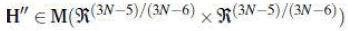finally is diagonalized to reveal its eigenvalues and vectors. For quite all systems, results obtained in both ways, with the above method and without it were compared. The results are very close to each other. The numerically optimized structures are almost exact and/or the Hessian matrix changes very little around the minimum and the numerical error can be ignored, using an appropriate method. Applying the new method in our further calculations, we were able to find positive semi definite Hessian matrices H’’ for all structures.

Calculation of numerical force constants (fcs) and vibrational frequency

A re-optimized structure of the force constants (FCs) could be extracted from the already optimized structure  as the following, the force(s) expressions were obtained by derivation of the energy expression (or) from the expression of energy, the forces can be easily calculated by derivation. Here, the Force(s) Fj that act on the j-th atom of the system can be calculated applying the Hellmann-Feynman theorem [20,21], so the forces are given as(28)

These are all identical to 0 (within numerical accuracy) for the optimized structure . Interatomic forces can easily be derived from an exact calculation of the gradients of the total energy at the considered atoms site, finally, the forces acting on an atom at Rj are obtained as follows:(29)

In our case, we have calculated as the numerical first-order derivatives of the forces instead of the numerical-second-order derivatives of the total energy. In principle there is no difference, but numerically the approach of using the forces is more accurate and, moreover, it requires much less calculation. However, to extract the force constants (FCs) in a atomic cluster directly, rather than indirectly through the agency of energy, a finite difference formula has been introduced as following.

We obtained our results by using the following formula,(30)(31)

So for convenience eqn. (30 and 31) can be written as,(32)(33)

for homonuclear case, M represents the atomic mass,(34)

When the forces on atoms are known, the FCs can be used to compute relaxations and relaxation energies. In total we end up with (3N×3N) values. The complete list of these force constants (FCs) is called the Hessian Hi j, which is a (3N _3N) matrix. Here, i is the component of (x, y or z) of the force on the j’th atom, so we get 3N. DF is the average difference of the two first derivatives of the force constants (FCs) and ds is a small displacement within the nuclear coordinates. We found that a PES over which the gradients extend for a small displacements ds = ±[0:01] a.u. of equilibrium coordinate value of cluster, which is a reasonable value and allowed to discriminate between the translational, rotational motion (Zeroeigenvalues) and the vibrational motion [i is vibrational frequencies [(low(min), high(max))] (Non-Zero-eigenvalues) of the atoms (or molecules) of the Hessian eigenvalues.

The vibrational partition function

The vibrational partition function is calculated by normal mode analysis. The partition function yields all equilibrium thermal properties of the clusters and harmonic approximation was used in the calculation of the vibrational contribution to the cluster partition function. Reducing dimensionality can bring entirely new properties for the thermodynamics of vibrational states for nanoclusters. The one-dimensional vibrational partition function zvib expressed by a sum over all possible vibrational states of the system,(35)

kB is Boltzmann’s constant, T is the absolute temperature and Ei is the energy corresponding to the vibrational state i. Each vibrational state of the system consisting of (3N-5)/ (3N-6) harmonic oscillators which are by construction linearly independent. The partition function of a cluster is evaluated in the same way one would evaluate that of a polyatomic molecule. The energy of cluster or molecule is assumed to be separable , i.e.,(36)

will influence each other. For example, eqn. (35) implies that the curvature of the potential energy surface of the electronically excited molecule is the same as for the molecule in its electronic ground state. This is a necessary condition for the vibrational frequencies and therefore for the vibrational energy to be independent from the electronic state of the molecule. Also, if the energy surfaces of the ground state and an electronically excited state come close together (avoided crossing), the separability of the electronic and vibrational modes may be a poor approximation (breakdown of the Born-Oppenheimer approximation) . Similarly, rotational excitation will have an impact on the bond length of "floppy" (soft bending potential) molecules and subsequently on the vibrational levels .

However, it can be shown that, for sufficiently low temperatures, the coupling effects are small and can be neglected, because the molecule is not likely to be in a highly excited state, where coupling becomes important. As long as the vibrations can be treated within the harmonic-oscillator-normal-mode model, the anharmonicity of the potential can be neglected and the average bond lengths won’t increase with vibrational excitation. Under the assumptions implied by eqn. (35), we can re-write the molecular partition function in a factorized form,(37)

where the sum has to be performed over all combinations of vibrational, rotational, translational and electronic states(38)

But the above result is just the product of partition functions which only take into account a single mode of excitation, i.e.,(39)

This result shows that in the case of negligible coupling of the different excitations it is possible to approximate the partition function as a product of translational, rotational, vibrational and electronic partition functions.

The individual contributions

In the present work, we focus on the size and temperature dependence of the vibrational part of the heat capacity. It was demonstrated, that the approximations introduced by the harmonicoscillator-normal-mode model, which imply that the different vibrational modes can be treated independently from each other, lead to a factorization of the vibrational partition function itself. This work does not answer the question, whether the electrons are irrelevant for the thermodynamic quantities or not. Most often, the electronic excitation energies are much larger than Kt and we are going to neglect the electronic partition function. We still need to find expressions for the translational, the rotational and the vibrational partition functions, so as to get access to the partition function and subsequently to the heat capacity we seek. According to the above and to eqn. (38), as an enabling the cluster partition function to be written as a product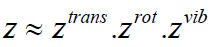(40)

In the literature, approximate formulae for the translational and the rotational part of the partition function are derived . The translational part ztrans is related to center of mass translation and can approximatively be treated within the ideal-gas model. An approximate formula for the rotational part zrot is found by application of the concepts of a rigid rotator on the cluster’s structure. However, based on the equipartition theorem in classical statistical thermodynamics, we know that in the case of the classical limit, the internal energy of a system will distribute itself evenly among its quadratic degrees of freedom if not only the lowest corresponding energy levels are significantly populated, but also the higher ones. The population of the quantum states of a given degree of freedom is temperature dependent. The closer the energies of the different quantum states lie together, the more probable it is to find a molecule in an excited state and the lower will be the temperature for which the classical limit is reached and the results of the equipartition theorem can be applied. It can easily be shown that the energy differences between the rotational and the translational states of a typical system are small compared to kT [24-32]. The equipartition theorem states, that each degree of freedom receives an average energy of 1/2kT. Since translation and rotation (of a nonlinear molecule) correspond to six degrees of freedom, the contribution to the internal energy is 3kT. In the present application, the translational and the rotational contributions are treated according to the theorem described above and only the vibrational partition function will be considered rigorously. In the case of the classical limit, the rotational and translational parts of the partition function do not depend on the cluster structure. Since the (3N-6) oscillators are by construction linearly independent, the vibrational energy can be expressed as a sum of the vibrational energies of each independent mode, i.e.,(41)

NVM being the number of normal vibrational modes of the cluster. The vibrational partition function Zvib expressed by a sum over all possible vibrational states of the system,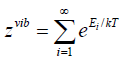(42)

Ei is the energy corresponding to the vibrational state i. Each vibrational state of the system consisting of (3N-6) harmonic oscillators is defined by a distinct set {nj | j = 1,2,…,(3N-6)} of (3N- 6) vibrational quantum numbers,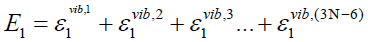(43)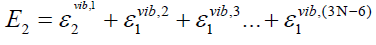(44)(45)

harmonic oscillator and the lower index specifies the quantum state it is in. For our understanding, we can write(46)

And again, rearranging the terms in the above equation yields a factorized vibrational partition function, i.e., a product of the (3N-6) partition functions each of which describes one individual harmonic oscillator,(47)

Recalling the energy states of the harmonic oscillator(48)

we are now in the position to give a concrete expression for the vibrational partition function. Given ωi, the angular frequency of the i-th harmonic oscillator, the partition function describing it is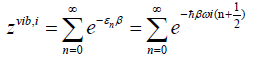(49)

where we introduced the inverse temperature ß=1/kT. Rearranging (48) leads us to(50)(51)

The contributions of translation and rotation depend only on the mass and moment of inertia of the molecule, and they are thus easy to calculate using the simple models of the particle in a box and the rigid rotator, respectively. In contrast, the vibrational contributions are, in general, difficult to evaluate, and thus are the prime issue of this work. The individual terms in the product are evaluated from standard statistical mechanical formulas (using the harmonic approximation) to evaluate the vibrational component zvib, Combining the above result with (46), we finally see, that(52)

NVM is the number of normal vibrational modes of the cluster. The above calculations were used to examine the helmholtz free energy of formation of the clusters as a function of temperature.

#### Results and Discussion

In this article, we present only about the symmetric structure of the gold atomic neutral clusters (Au3-20) and its vibrational spectrum. The predicted spectrum ranges were found to be in a range of 0.55 to 370.72 cm-1. By using a parameterized tightbinding density-functional method combined with numerical finite difference method we have confirmed the global total energy-minimum structures for gold clusters containing up to 20 atoms. The calculated vibrational frequency ranges were tabulated (Tables 1-18). Interestingly, we have observed some double and triple state degeneracy at T= 0. In fact, Au6-8 clusters are unique among the other clusters, due to their degeneracy nature. Thus as a different molecule with atomic packing could be similar to that of bulk gold but with very different properties that is with respect to the electron density between the different concentric layers. However, non-degenerate modes, because of their higher symmetry, are easier to visualize at the spectrum. Interestingly, the lower non-degenerate mode displaces atoms only at the edges, not at the vertices or face centers. Since different symmetry gives rise to a large number of degenerate modes, but only some distinct modes frequencies are expected for the rest of the clusters. Furthermore, normal modes of vibration are having both infrared and Raman-active, and the remains are optically silent (which will have some experimental difficulties). Nevertheless, all interactions may be accompanied by electron transfer and the interactions onto the vertex, edge, or inner gold atoms. Density Functional calculations predict that Au3-20 possesses a different geometry structures which were then verified through the Gabedit package  (Tolerance for principal axis classification: 0.00500 in angstrom (Å) and Precision for atom position: 0.09399 in angstrom (Å)). In addition to that the predicted minima of the global structure optimization of Au3 to Au20 were plotted in Figures 1-6 by increasing cluster size and energy at T = 0 K. Overall, cluster size, spectrum ranges and the symmetry of gold clusters from N=3 to 20 atoms are also mentioned at the Table 19.

Normal Vibrational
Modes (NVM=3)
Vibrational frequency (ωi) in cm1
1 19.21
2 87.47
3 246.21

Table 1: Calculated vibrational frequency (ωi) of the re-optimized gold cluster, Au3 at T=0.

Normal Vibrational Modes (NVM=6) Vibrational frequency (ωi) in cm1
1 9.83
2 31.63
3 55.2
4 98
5 147.12
6 165.46

Table 2: Calculated vibrational frequency (ωi) of the re-optimized gold cluster, Au4 at T=0.

Normal Vibrational Modes (NVM=9) Vibrational frequency (ωi) in cm1
1 0.55
2 2.5
3 43.25
4 47.7
5 81.82
6 132.74
7 196.33
8 224.53
9 276.83

Table 3: Calculated vibrational frequency (ωi) of the re-optimized gold cluster, Au5 at T=0.

Normal Vibrational Modes (NVM=12) Vibrational frequency (ωi) in cm 1
1 2.44
2 2.44
3 2.44
4 38.59
5 38.59
6 58.51
7 58.51
8 118.06
9 164.08
10 178.3
11 282.99
12 282.99

Table 4: Calculated vibrational frequency (ωi) of the re-optimized gold cluster, Au6 at T=0.

Normal Vibrational Modes (NVM=15) Vibrational frequency (ωi) in cm1
1 20.48
2 20.48
3 40.78
4 55.5
5 55.5
6 58.63
7 58.63
8 106.38
9 106.38
10 109.62
11 142.05
12 142.05
13 214.62
14 214.62
15 235.19

Table 5: Calculated vibrational frequency (ωi) of the re-optimized gold cluster, Au7 at T=0.

Normal Vibrational Modes (NVM=18) Vibrational frequency (ωi) in cm1
1 4.66
2 4.67
3 24.52
4 24.53
5 24.53
6 64.97
7 67.92
8 67.92
9 67.92
10 102.72
11 102.72
12 102.72
13 131.45
14 131.45
15 198.97
16 215.07
17 215.08
18 215.08

Table 6: Calculated vibrational frequency (ωi) of the re-optimized gold cluster, Au8 at T=0.

Normal Vibrational Modes (NVM=21) Vibrational frequency (ωi) in cm1
1 2.76
2 8.29
3 10.32
4 43.42
5 48.78
6 59.99
7 64.54
8 73.24
9 92.68
10 99.4
11 102.32
12 119.75
13 133.5
14 137.53
15 168.78
16 171.32
17 173.67
18 181.97
19 204.4
20 220.93
21 313.24

Table 7: Calculated vibrational frequency (ωi) of the re-optimized gold cluster, Au9 at T=0.

Normal Vibrational Modes (NVM=24) Vibrational frequency (ωi) in cm1
1 34.18
2 35.73
3 40.94
4 42.96
5 47.34
6 53.51
7 54.63
8 56.61
9 73.12
10 117.24
11 119.14
12 131.3
13 135.27
14 137.72
15 141.02
16 158.26
17 162.84
18 197.3
19 201.95
20 208.66
21 211
22 219.66
23 225.22
24 341.88

Table 8: Calculated vibrational frequency (ωi) of the re-optimized gold cluster, Au10 at T=0.

Normal Vibrational Modes (NVM=27) Vibrational frequency (ωi) in cm1
1 7.32
2 13.99
3 23.32
4 25.54
5 26.82
6 31.14
7 38.99
8 40.47
9 47.89
10 50.7
11 58.37
12 77.1
13 89.59
14 99.44
15 105.82
16 116.72
17 139.15
18 140.83
19 155
20 167.69
21 183.43
22 205.44
23 216.68
24 225.77
25 263.82
26 272.52
27 291.85

Table 9: Calculated vibrational frequency (ωi) of the re-optimized gold cluster, Au11 at T=0.

Normal Vibrational Modes (NVM=30) Vibrational frequency (ωi) in cm1
1 1.01
2 12.39
3 15.81
4 19.28
5 25.17
6 25.55
7 29.87
8 31.54
9 33.8
10 39.88
11 42.25
12 54.2
13 67.07
14 82.31
15 83.87
16 100.68
17 104.66
18 117.65
19 127.19
20 138.92
21 149.36
22 153.11
23 172.5
24 182.26
25 187.53
26 205.84
27 233.56
28 245.6
29 264.76
30 325.89

Table 10: Calculated vibrational frequency (ωi) of the re-optimized gold cluster, Au12 at T=0.

Normal Vibrational Modes (NVM=33) Vibrational frequency (ωi) in cm1
1 11.45
2 13.66
3 14.02
4 20.81
5 25.53
6 26.48
7 28.3
8 32.12
9 32.79
10 34.11
11 37.69
12 41.07
13 53.72
14 56.3
15 65.49
16 69.53
17 84.35
18 94
19 100.49
20 117.37
21 128.98
22 134.62
23 145.48
24 147.39
25 172.04
26 185.62
27 200.02
28 210.07
29 214.17
30 225.57
31 242.58
32 263.26
33 334.7

Table 11: Calculated vibrational frequency (ωi) of the re-optimized gold cluster, Au13 at T=0.

Normal Vibrational Modes (NVM=36) Vibrational frequency (ωi) in cm1
1 17.02
2 18.86
3 19.53
4 19.75
5 24.31
6 25.34
7 27.18
8 34.04
9 34.53
10 38.67
11 42.79
12 43.21
13 44.63
14 58.46
15 58.89
16 69.39
17 70.86
18 84.22
19 84.87
20 92.74
21 109.7
22 113.59
23 130.96
24 132.76
25 147.38
26 152.9
27 167.18
28 176.74
29 181.82
30 182.93
31 186.81
32 198.47
33 203.39
34 215.87
35 225.76
36 240.2

Table 12: Calculated vibrational frequency (ωi) of the re-optimized gold cluster, Au14 at T=0.

Normal Vibrational Modes (NVM=39) Vibrational frequency (ωi) in cm1
1 7.49
2 12.27
3 17.09
4 22.53
5 23.59
6 31.08
7 34.1
8 42.3
9 47.22
10 48.84
11 60.25
12 67.56
13 69.45
14 73.36
15 74.69
16 80.68
17 90.49
18 92.83
19 96.03
20 102.7
21 106.87
22 112.73
23 119.17
24 136.82
25 137.6
26 148.91
27 151.87
28 162.23
29 168.74
30 177.56
31 190.79
32 200.3
33 202.73
34 207.81
35 218.03
36 231.37
37 238.11
38 282.44
39 285.36

Table 13: Calculated vibrational frequency (ωi) of the re-optimized gold cluster, Au15 at T=0.

Normal Vibrational Modes (NVM=42) Vibrational frequency (ωi) in cm1
1 17.13
2 21.26
3 22.19
4 30.59
5 31.41
6 36.5
7 40.37
8 43.02
9 46.15
10 49.37
11 56.35
12 57.66
13 58.24
14 66.67
15 75.28
16 77.28
17 83.12
18 85.97
19 89.07
20 99.59
21 110.56
22 116.51
23 120.99
24 131.27
25 142.26
26 155.39
27 155.74
28 180.46
29 183.1
30 185.17
31 190.34
32 192.71
33 204.67
34 215.89
35 219.11
36 233.45
37 252.64
38 261.57
39 269.35
40 272.28
41 285.58
42 295.76

Table 14: Calculated vibrational frequency (ωi) of the re-optimized gold cluster, Au16 at T=0.

Normal Vibrational Modes (NVM=45) Vibrational frequency (ωi) in cm1
1 9.57
2 10.23
3 11.81
4 12.98
5 16.73
6 18.47
7 20.67
8 21.59
9 24.78
10 26.02
11 26.95
12 31.39
13 32.96
14 38.97
15 45.72
16 50.75
17 58.65
18 59.62
19 62.98
20 65.27
21 77.5
22 78.84
23 84.16
24 88.4
25 99.36
26 104.69
27 112.87
28 119
29 132.83
30 148.1
31 155.52
32 159.12
33 168.13
34 181.59
35 183.53
36 187.54
37 194.25
38 201.8
39 214.28
40 221.91
41 228.4
42 232.36
43 259.35
44 262.1
45 302.78

Table 15 Calculated vibrational frequency (ωi) of the re-optimized gold cluster, Au17 at T=0.

Normal Vibrational Modes (NVM=48) Vibrational frequency (ωi) in cm1
1 8.07
2 8.72
3 10.91
4 11.44
5 18.62
6 19.51
7 21.91
8 22.69
9 24.18
10 25.68
11 29.03
12 29.49
13 33.01
14 34.44
15 43.77
16 45.21
17 47.98
18 49.64
19 50.56
20 51.67
21 53.09
22 54.76
23 71.03
24 92.08
25 97.28
26 98.78
27 104.19
28 110.32
29 114.81
30 126.82
31 133.16
32 137.37
33 141.75
34 142.98
35 156.32
36 161.96
37 166.18
38 179.03
39 180.81
40 184.77
41 194.95
42 200.6
43 201.99
44 207.14
45 216.2
46 219.78
47 226.16
48 232.46

Table 16: Calculated vibrational frequency (ωi) of the re-optimized gold cluster, Au18 at T=0.

Normal Vibrational Modes (NVM=51) Vibrational frequency (ωi) in cm1
1 10.08
2 10.56
3 11.65
4 12.34
5 13.38
6 16.33
7 17.71
8 19.64
9 21.8
10 23.16
11 24.55
12 26.88
13 27.88
14 30.38
15 33.34
16 35.09
17 39.16
18 40.95
19 43.72
20 48.42
21 50.95
22 59.77
23 65.39
24 70.89
25 73.07
26 79.22
27 87.85
28 92.93
29 95.07
30 113
31 116.27
32 124.88
33 131.43
34 137.07
35 144.15
36 152.87
37 158.47
38 165.28
39 175.5
40 177.26
41 188.13
42 193.74
43 205.81
44 208.33
45 213.7
46 229.36
47 238.39
48 256.6
49 284.77
50 294.91
51 319.18

Table 17: Calculated vibrational frequency (ωi) of the re-optimized gold cluster, Au19 at T=0.

Normal Vibrational Modes (NVM=54) Vibrational frequency (ωi) in cm1
1 3.99
2 11.21
3 13.66
4 16.56
5 18.27
6 19.12
7 19.57
8 22.74
9 25.62
10 26.45
11 27.7
12 29.32
13 32.06
14 34.14
15 38.18
16 39.7
17 44.58
18 48.75
19 49.61
20 51.26
21 61.33
22 63.22
23 68.05
24 69
25 73.78
26 84.34
27 85.68
28 89.1
29 94.14
30 95.75
31 102.16
32 103.33
33 122.9
34 130.44
35 136.72
36 140.64
37 148.64
38 155.61
39 160.73
40 165.07
41 172.09
42 177.76
43 186.66
44 195.13
45 203.18
46 209.21
47 213.65
48 222.34
49 227.54
50 247.44
51 253.04
52 267.51
53 276.35
54 370.72

Table 18: Calculated vibrational frequency (ωi) of the re-optimized gold cluster, Au20 at T=0.

Figure 1: Predicted minima of the global structure optimization of Au3 (C2v), Au4 (D2h) and Au5 (C2v) (from top to bottom) by increasing cluster size and energy at T=0 K.

Figure 2: Predicted minima of the global structure optimization of Au6 (D3h), Au7 (D5h) and Au8 (Td ) (from top to bottom) by increasing cluster size and energy at T=0 K.

Figure 3: Predicted minima of the global structure optimization of Au9 (Cs), Au10 (S8) and Au11 (C1) (from top to bottom) by increasing cluster size and energy at T=0 K.

Figure 4: Predicted minima of the global structure optimization of Au12 (C1), Au13 (C1) and Au14 (Cs) (from top to bottom) by increasing cluster size and energy at T=0 K.

Figure 5: Predicted minima of the global structure optimization of Au15 (C1), Au16 (Cs) and Au17 (C1) (from top to bottom) by increasing cluster size and energy at T=0 K.

Figure 6: Predicted minima of the global structure optimization of Au18 (D1),Au19 (C1) and Au20 (C1) (from top to bottom) by increasing cluster size and energy at T=0 K.

Cluster Size (N)   Spectrum Range (T=0 K) in cm-1 Symmetry (Theoretical (Gabedit package))25 Symmetry (Theoretical)13
Au3 19.21 - 246.21 C2v D2
Au4 09.83 - 165.46 D2h D2h
Au5 00.55 - 276.83 C2v C2v
Au6 02.44 - 282.99 D3h D3h
Au7 20.48 - 235.19 D5h D5h
Au8 04.66 - 215.08 Td Td
Au9 02.76 - 313.24 Cs D2v=D2
Au10 34.18 - 341.88 S8 D2
Au11 07.32 - 291.85 C1 Cl
Au12 01.01 - 325.89 C1 Cl
Au13 11.45 - 334.70 C1 Cs
Au14 17.02 - 240.20 Cs Cs
Au15 07.49 - 285.36 C1 Cl
Au16 17.13 - 295.76 Cs Cs
Au17 09.57 - 302.78 C1 Cl
Au18 08.07 - 232.46 D1 C2
Au19 10.08 - 319.18 C1 Cl
Au20 03.99 - 370.72 C1 Cl

Table 19: Size and Symmetry of gold clusters from N=3 to 20 atoms.

#### Conclusion

We have extracted vibrational frequency of the re-optimized gold atomic clusters (Au3��20) at temperature T=0 K by using DFTB method. The present calculations of the frequency spectrum is a predictions to be confirmed when the experimental data become available. The Hessian matrix, calculated to obtain the normal modes of of vibration in this work, can be much useful in MD simulations which can find a dependency of the gold-atomicclusterassisted catalytic process on temperature. We have observed vibrational properties of the clusters in order to explore the interaction between stability and the structure of clusters. Our new approach is worthy of further investigation and would pave a way in realizing numerical values which would allow for an experimental vibrational spectrum, which would prove crucial in development of nanoelectronic devices. Nevertheless, our work gives a possible cause for the size and structure effect of Au atomic clusters.

#### Dedication

Dedicated to Professor Prasanta Kumar Panigrahi, IISER, Kolkata, India, Professor Michael Springborg, University of Saarland, Germany, on the occasion of their 60th birthday, and Professor Kwang Soo Kim, UNIST, S. Korea, on the occasion of his 67th birthday.

#### Acknowledgements

A part of this work was supported by the German Research Council (DFG) through project Sp 439/23-1. We gratefully acknowledge their very generous support.